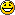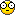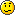# Thread: Inertial guidance system simplified

1. ## Inertial guidance system simplified

If you understand this and can explain it to me I'll buy you a beer in AmsterdamThe aircraft knows where it is at all times. It knows this because it knows where it isn't.
By subtracting where it is from where it isn't, or where it isn't from where it is (whichever is the greater), it obtains a difference, or deviation.The Inertial Guidance System uses deviations to generate error signal commands which instruct the aircraft to move from a position where it is to a position where it isn't, arriving at a position where it wasn't, or now is. Consequently, the position where it is, is now the position where it wasn't; thus, it follows logically that the position where it was is the position where it isn't. In the event that the position where the aircraft now is, is not the position where it wasn't, the Inertial Guidance System has acquired a variation. Variations are caused by external factors, the discussions of which are beyond the scope of this report.A variation is the difference between where the aircraft is and where the aircraft wasn't. If the variation is considered to be a factor of significant magnitude, a correction may be applied by the use of the autopilot system. However, use of this correction requires that the aircraft now knows where it was because the variation has modified some of the information which the aircraft has, so it is sure where it isn't. Nevertheless, the aircraft is sure where it isn't (within reason) and it knows where it was. It now subtracts where it should be from where it isn't, where it ought to be from where it wasn't (or vice versa) and intergrates the difference with the product of where it shouldn't be and where it was; thus obtaining the difference between its deviation and its variation, which is variable constant called "error".Reply With Quote

2. Ummmm... Ya I got this!!! NOT!!!!Reply With Quote

3. I seeeeee!!! this explains it all!If you understand this and can explain it to me I'll buy you a beer in Amsterdam
Where it most probably isn't....

AartReply With Quote

4. ## Re: Inertial guidance system simplified

The aircraft knows where it is at all times. It knows this because it knows where it isn't.
Actually it's quite simple, the answer is as it says in the above statement, the rest is just an explanation of how the above works.

This is not a new technique, this logic is used in robots.... well.... maybe new for the aeronautical industry. It's much more accurate to find out where you are by eliminating where you're not. With conventional avionics, including GPS, it calculates where you are by direct signals. Where as the above method is a process of elimination; if you are not here or there or the other place and so on...... then in the final computation, "You must be here." Rather relying on 1,2,or 3 signals you're location is computed on quite a bit more resources, all though it takes much more computer power to calculate, it's much more accurate.

I don't know if this helps or not.....Reply With Quote

5. Hello Matt... And yes it does make sense when you explain it that way!! THANKS!!!Reply With Quote

6. ## Beer in Leiden

Well Aart, I'll buy you a beer in Leiden....if you prefer this to AMSReply With Quote

7. Well Aart, I'll buy you a beer in Leiden....if you prefer this to AMS
Good idea!! We could drink it in my cockpit, welcome anytime!

AartReply With Quote

8. ## Local Meet and Greet

Sure would like to make a flight together in your cockpit, or watch you fly while I sip my beer.
Let me know when it would suit you by mail.
Have fun!Reply With Quote

9. ## Re: Inertial guidance system simplified

... By subtracting where it is from where it isn't, or where it isn't from where it is

...which also explains why I am insaneReply With Quote